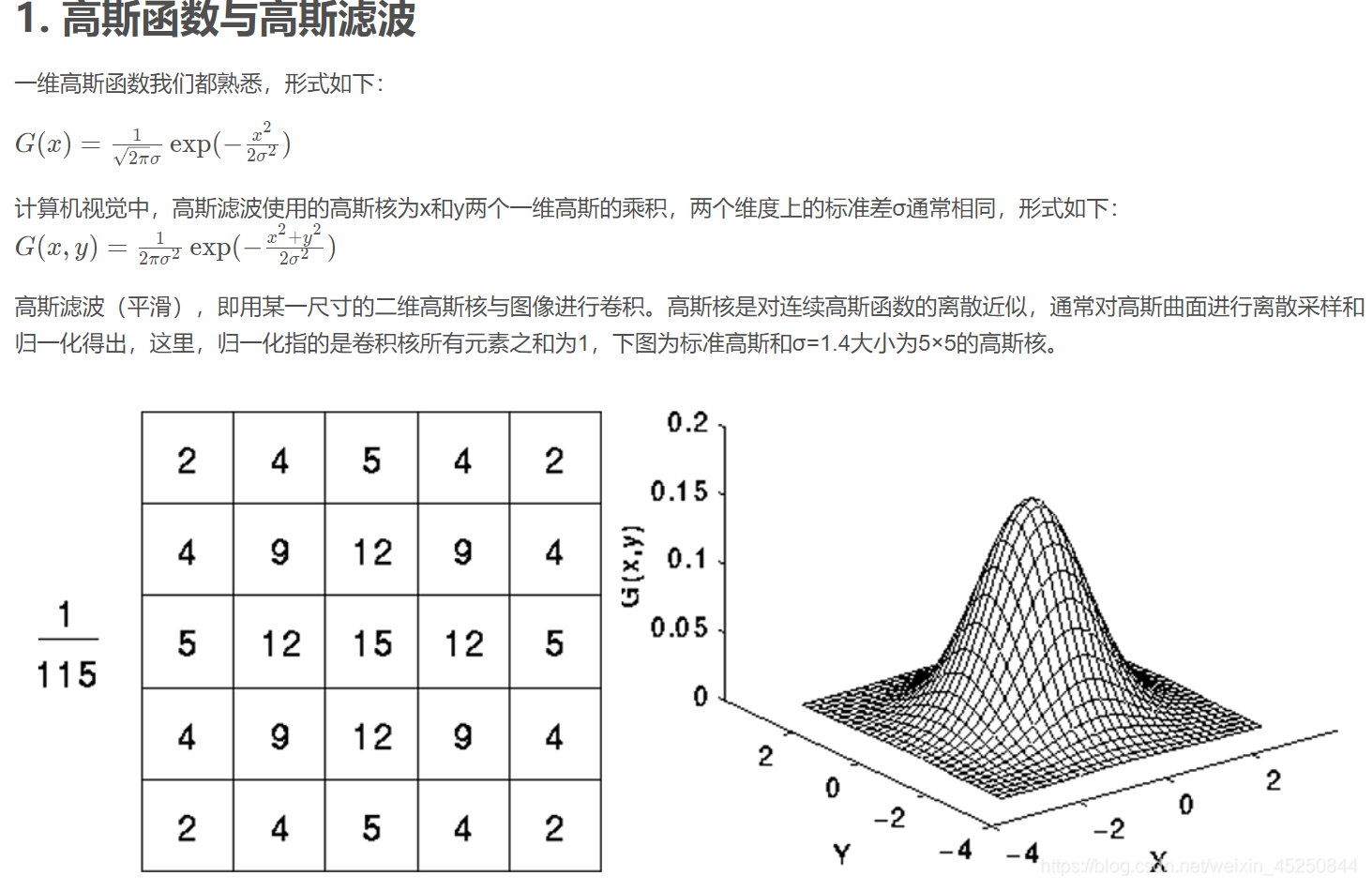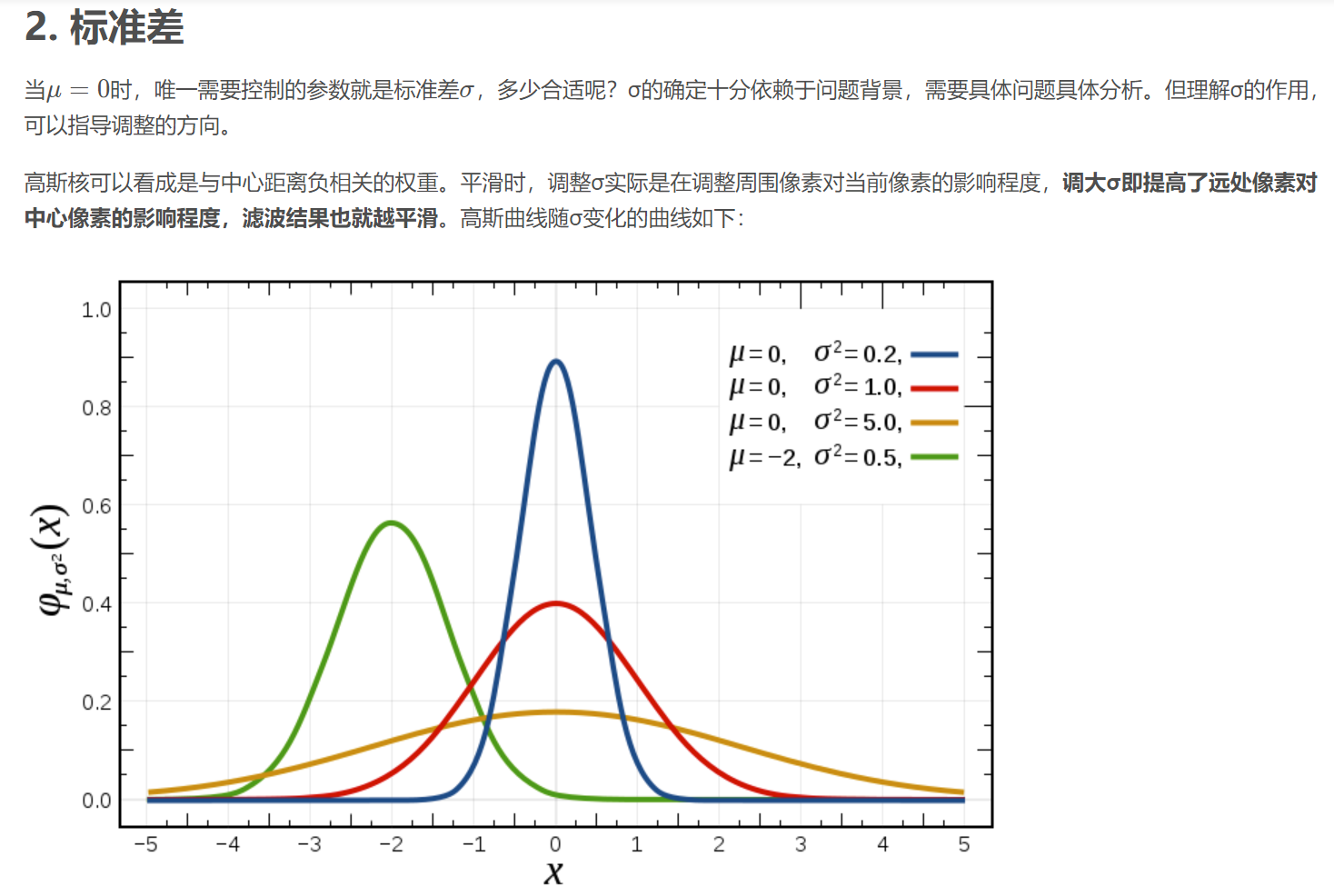# 前言

randn(m,n)就是产生服从方差为1，均值为0 的Gaussian分布的m*n大小的随机噪音矩阵。

# 一、高斯函数是什么？# 二、randn 产生 高斯噪声

randn(m,n)就是产生服从方差为1，均值为0 的Gaussian分布的mXn随机噪音矩阵，

noise=5+0.05*randn(m,n)

t=imread('E:\matlab DMP\lenaG.bmp');
[m,n,z]=size(t);
y=0+20*randn(m,n);%二维高斯分布矩阵 0是均值 20是标准差
y=uint8(y);
%加上噪声
t1=t+y;
subplot(1,2,1),imshow(t),title('原图');
subplot(1,2,2),imshow(t1),title('加入均值为0，标准差为20的高斯噪声后');

# 结果